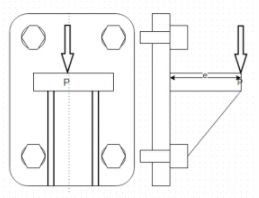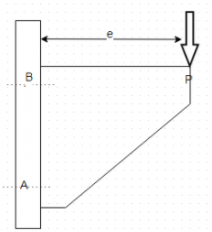Courses

# Test: Eccentric Loaded Perpendicular To Axis Of Bolt

## 10 Questions MCQ Test Machine Design | Test: Eccentric Loaded Perpendicular To Axis Of Bolt

Description
This mock test of Test: Eccentric Loaded Perpendicular To Axis Of Bolt for Mechanical Engineering helps you for every Mechanical Engineering entrance exam. This contains 10 Multiple Choice Questions for Mechanical Engineering Test: Eccentric Loaded Perpendicular To Axis Of Bolt (mcq) to study with solutions a complete question bank. The solved questions answers in this Test: Eccentric Loaded Perpendicular To Axis Of Bolt quiz give you a good mix of easy questions and tough questions. Mechanical Engineering students definitely take this Test: Eccentric Loaded Perpendicular To Axis Of Bolt exercise for a better result in the exam. You can find other Test: Eccentric Loaded Perpendicular To Axis Of Bolt extra questions, long questions & short questions for Mechanical Engineering on EduRev as well by searching above.
QUESTION: 1

### The following data is given for bracket P=20kN, e=100mm, S(yt)=400N/mm², FS=3Q. Find the direct shear stress in bolts if diameter =18mm

Solution:

Explanation: Direct shear stress=one fourth of P/A.

QUESTION: 2

### The following data is given for bracket P=20kN, e=100mm, S(yt)=400N/mm², FS=3Q. If distance between the bolts from the axis of tilting is 125mm and 25mm, then find the effective tensile force acting on the bolts lying at 125mm from tilting axis.

Solution:

Explanation: Tensile force=Pe x 125/2(125²+25²).

QUESTION: 3

### The following data is given for bracket P=20kN, e=100mm, S(yt)=400N/mm², FS=3Q. If distance between the bolts from the axis of tilting is 125mm and 25mm, then find the effective tensile force acting on the bolts lying at 25mm from tilting axis.

Solution:

Explanation: Tensile force=Pe x 25/2(125²+25²).

QUESTION: 4

The following data is given for bracket
P=20kN, e=100mm, S(yt)=400N/mm², FS=3Q. Which bolt can be used as criteria for designing?

Solution:

Explanation: The bolts farthest from the tilting axis are under the maximum tensile force.

QUESTION: 5

The following data is given for bracket
P=20kN, e=100mm, S(yt)=400N/mm², FS=3Q. Find the principal shear stress in the top bolts in terms of area A?

Solution:

Explanation: Shear Stress in one bolt=5000/A and tensile stress in upper bolt=7692.3/A. τ(max)=√[(σ/2)²+ τ²].

QUESTION: 6

The following data is given for bracket
P=20kN, e=100mm, S(yt)=400N/mm², FS=3Q. Find the size of the bolt using maximum shear stress theory.

Solution:

Explanation: 400 x 0.5/3 =6308.2 x 4 /πd².

QUESTION: 7

The following data is given for bracket
P=20kN, e=100mm, S(yt)=400N/mm², FS=3Q.  Find the size of the bolt using maximum principal stress theory.

Solution:

Explanation: σ(max)=σ/2 + √[(σ/2)²+ τ²]=10154.35/A = 35N/mm².

QUESTION: 8

A wall bracket is attached to the wall by means of 2 identical bolts, one at A and one at B. The force of 20kN acts at a distance of 400mm. Bolt A lies at a distance of 50mm from axis of tilt and bolt B 500mm.
Allowable shear stress in the bolts is 100N/mm² and tensile is 40N/mm².Q. Which bolt is used a criterion for designing?

Solution:

Explanation: The bolt lying farthest from the axis of tilt experience the maximum stress.

QUESTION: 9

A wall bracket is attached to the wall by means of 2 identical bolts, one at A and one at B. The force of 20kN acts at a distance of 400mm. Bolt A lies at a distance of 50mm from axis of tilt and bolt B 500mm.
Allowable shear stress in the bolts is 100N/mm² and tensile is 40N/mm².Q. Find the effective tensile force acting in the two bolts.

Solution:

Explanation: F₁=Pel₁/2(l₁² + l₂²) and F₂= Pel₂/2(l₁² + l₂²).

QUESTION: 10

A wall bracket is attached to the wall by means of 2 identical bolts, one at A and one at B. The force of 20kN acts at a distance of 400mm. Bolt A lies at a distance of 50mm from axis of tilt and bolt B 500mm.
Allowable shear stress in the bolts is 100N/mm² and tensile is 40N/mm².Q. Find the radius of Mohr circle which can be made from the shear stress and tensile stress acting on bolts.(Consider bolt under max stress and area of bolt is A)

Solution:

Explanation: r=√(σ/2)²+ τ² where σ=7920.8/A and τ=20000/4A.